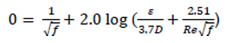## Find a friction factor-newton-raphson method, Chemical Engineering

Assignment Help:

Colebrook equation is used to find a friction factor, f, in a pipe flow as given by:where ? and Re are the roughness ratio and Reynolds number respectively. Considering air flow through a pipe with a diameter of 0.005m, ?=0.0015 mm, and Re=13,743, compute the friction number using the Newton-Raphson method and the Matlab built-in function "fzero".

report

au

#### Convection heat transfer, An enclosure haves Helium at a pressure of 1.3 at...

An enclosure haves Helium at a pressure of 1.3 atm and has two vertical heating surfaces, which are keeps at 80 and 20 oC respectively. The vertical surfaces are 40x40 cm and are s

#### INORGANIC CHEMISTRY, DEFINE INORGANIC CHEMISTRY

DEFINE INORGANIC CHEMISTRY

#### Separation processes, What kind of separation processes are there?

What kind of separation processes are there?

#### Calculate the pressure and composition of the vapor phase, For the binary s...

For the binary system 2, 4-dimethylpentane (1) + benzene (2) the excess Gibbs energy of the liquid phase is given by: G E /(x 1 x 2 ) = (A 12 x 1 + A 21 x 2 ) a. Calculate t

#### #titSTEAM GENERATION AT CONSTANT PRESSUREle.., #question.WHAT IS STEAM GENE...

#question.WHAT IS STEAM GENERATION AT CONSTATNT PRESSURE .

#### #titleHardness of Water, Ask question #Minimum 100 words accepted.hardness ...

Ask question #Minimum 100 words accepted.hardness of bore water is less than that of sea water-justify

#### 20TPD Mg(oh)2 by brine, 20TPD Mg(oh)2 by brine

20TPD Mg(oh)2 by brine

#### Requirment for study design and features of laboratory, REQUIREMENTS Wo...

REQUIREMENTS Workbook cum Lab. manual Block 1 (LT-1 course) Pencil Pen Eraser Scale Coloured pens